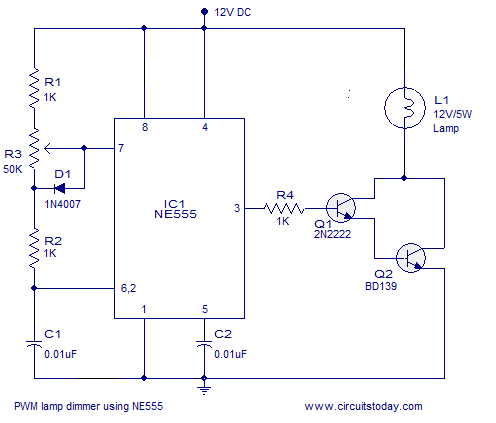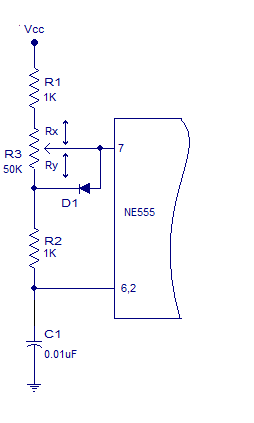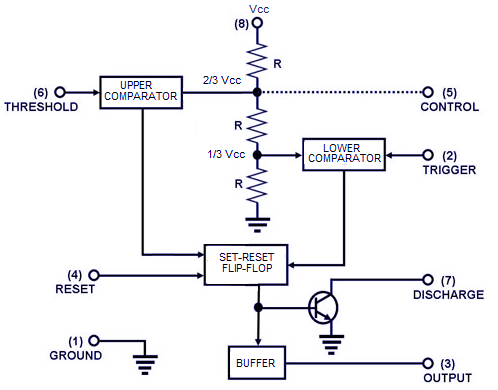## Audio

[Audio][slideshow]

### PWM lamp dimmer.

A simple and efficient PWM lamp dimmer using timer IC NE555 is discussed in this article. Yesterdays linear regulator based dimmers can only attain a maximum efficiency of 50% and are far inferior when compared to the PWM based dimmers which can hit well over 90% efficiency. Since less amount of power is wasted as heat, the switching elements of PWM dimmers require a smaller heat sink and this saves a lot of size and weight. In simple words, the most outstanding features of the PWM based lamp dimmers are high efficiency and low physical size. The circuit diagram of a 12V PWM lamp dimmer is shown below.

We recommend 3 very good books to learn the basics and applications of the 555 timer IC. These books have been thoroughly reviewed and they can be purchased by clicking on this link:- 3 Great Books to Learn 555 Timer Circuits and ProjectsFig 1 : PWM lamp dimmer using NE555

As you can see, NE555 timer IC which is wired as an astable multivibrator operating at 2.8KHz forms the heart of this circuit. Resistors R1,R2, POT R3 and capacitor C1 are the timing components. Duty cycle of the IC’s output can be adjusted using the POT R3. higher the duty cycle means higher the lamp brightness and lower the duty cycle means lower the lamp brightness. Diode D1 by-passes the lower half of the POT R3 during the charging cycle of the astable multivibrator. This is done in order to keep the output frequency constant irrespective of the duty cycle. Transistors Q1 and Q2 forms a darlington driver stage for the 12V lamp. Resistor R4 limits the base current of transistor Q1.

#### Understanding the variable duty cycle astable multivibrator.

As I have said earlier, the variable duty cycle astable multi vibrator based on NE555 forms the foundation of this circuit and a good knowledge on it is essential for designing projects like this. For the ease of explanation the timing side of the astable multivibrator is redrawn in the figure below.Fig 2: Astable multivibrator with variable duty cycle

Upper and lower halves of the POT R3 are denoted as Rx and Ry respectively. Consider the output of the astable multivibrator to be high at the starting instant. Now the capacitor C1 charges through the path R1, Rx, and R2. The lower half of POT R3 ie; Ry is out of the scene because the diode D1 by-passes it. When the voltage across the capacitor reaches 2/3 Vcc, the internal upper comparator flips its output which makes the internal flip flop to toggle its output. As a result the output of the astable multivibrator goes low. In simple words, the output of the astable multivibrator remains high until the charge across C1 becomes equal to 2/3 Vcc and here it is according to the equation Ton =0.67(R1+Rx+R2)C1.

Since the internal flip flop is set now, the capacitor starts discharging through the path R2,Ry into the discharge pin. When the voltage across the capacitor C1 becomes 1/3 Vcc, the lower comparator flips its output and this in turn makes the internal flip flop to toggle its output again. This makes the output of the astable multivibrator high. To be simple, the output of the astable multivibrator remains low until the voltage across the capacitor C1 becomes 1/3 Vcc and it is according to the equation Toff = 0.67(R2+Ry)C1. Have a look at the internal block diagram of NE555 timer shown below for better understanding.Fig3: NE555 internal block diagram

##### How does the frequency remain constant irrespective of the position of POT3 knob?.

What ever may be the position of POT3 knob, the total resistance across it remains the same (50K here). If anything decreases in the upper side (Rx) the same amount will be increased in the lower (Ry) and the same thing gets applied to the higher(Ton) and lower(Toff) time periods. The derivation shown below will help you to grasp the matter easily.

With reference to Fig 2, we have:

Ton = 0.67(R1+Rx+R2)C1

Toff= 0.67(R2+Ry)C1

Total time period of the output waveform “T” is according to the equation :

T = Ton + Toff

There fore, T = 0.67(R1+Rx+R2+R2+Ry)C1

T= 0.67(R1+2R2+Rx+Ry)C1

We know that Rx+Ry = R3

There fore T = 0.67(R1+2R2+R3)C1

Therefore frequency F = 1/(0.67(R1+2R2+R3)C1)

From the above equation its is clear that the frequency depends only on the value of the components C1, R1, R2 and the over all value of R3 and it has nothing to do with the position of R3 knob.

[Lights][grids]